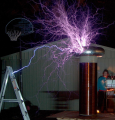# Inductive Spike Voltage?

#### imzack

Joined Nov 3, 2010
72
I am making a LARGE inductor. I want to experiment w/ charging it, and discharging it as fast as possible.

How would I go about figuring out how large of a inductive voltage spike I would get when I discharge a coil?

Thanks!

#### ronsimpson

Joined Oct 7, 2019
907
Inductive kick back, (discharge) is much like throwing a ball. At see level the earths gravity pulls the ball down and limits how high you can throw. Where I live (high altitude) the air effects the ball less and gravity is less. On the moon you can throw 6x higher. In space the ball will go to infinity.
There are many things that limit the inductive spike. Large might not be better.
What are you trying to do?#### crutschow

Joined Mar 14, 2008
25,388
How would I go about figuring out how large of a inductive voltage spike I would get when I discharge a coil?
It depends upon the coil inductance, the coil current, and how fast you want to start and stop the current.
The relation is V = L di/dt, where V is the voltage, L is the inductance in Henrys, and di/dt is the rate of current change in the inductor given in amps/second.

#### imzack

Joined Nov 3, 2010
72
I am trying to make a large electro-magnet. Though its a aircoil. (Need it to de-energize ASAP)

and V = L (di/dt)

L would be a constant... and assuming steady-state DC, the inductor would be a short, fully charged.

When the switch opens, we have a open circuit, but current though the inductor keeps flowing, building a large voltage potential across it. (As the charge has no-where to flow)

I just am having issues conceptualizing the 'switch off' time, and converting that into a voltage that I should be concerned about.

If I had a switch that could open up instantaneous, would that mean I would have a infinite voltage spike?

Should I design around the speed a FET can switch at, and then derive the max voltage based off worst case/fastest switch time of hardware I have at hand?

If I am on the right path, would I determine di/dt by taking the max current to min current, and determining the slope based on the switch time between these two points?

Anyone willing to walk me through the proper thought process?

Thanks!

#### imzack

Joined Nov 3, 2010
72

#### shortbus

Joined Sep 30, 2009
7,920
If I switch the circuit open, what would the slope look like?
A lot would depend on the voltage you charged it at, not the amperage. With it being an open circuit at the shutdown of charging where is the amps or volts going to go? If the volts are high enough you will get a spark across the air gap, but only if it is high enough to jump your air gap. Voltage jumps the gap not amperage.

#### Chris65536

Joined Nov 11, 2019
224
When the switch opens, we have a open circuit, but current though the inductor keeps flowing
I think the current goes instantly to zero here. With the switch open, how could it decay gradually? The voltage spikes, and then decays as the magnetic field dissipates. If the peak voltage is high enough, it can arc across the switch. I am interested, but I do not know how this voltage curve is calculated...

#### Bordodynov

Joined May 20, 2015
2,658
The inductive emission is determined by the energy stored in the inductance. This is EL=i^2*L/2. The second and main factor is the parasitic capacitance. It includes the capacity of the inductance itself (this capacity is always there). It also includes the parasitic capacitance of the switching element. In particular, it's the transistor's capacitance. When the circuit breaks, the inductance energy is pumped into the energy of the parasitic capacitor. The time of energy exchange is limited. This energy is equal to EC=V^2*Cpar/2. It follows from the formula that the smaller the capacity specified, the more voltage ejection can be obtained. It is possible that a switching element (e.g. transistor) will break down earlier.

•Chris65536

#### Chris65536

Joined Nov 11, 2019
224
When the circuit breaks, the inductance energy is pumped into the energy of the parasitic capacitor.
Excellent. Thank you for this explanation!

#### Orson_Cart

Joined Jan 1, 2020
18
the spike voltage is limited by the capacitance of the winding and the breakdown of the wire insulation ( or wire to core )

•Chris65536